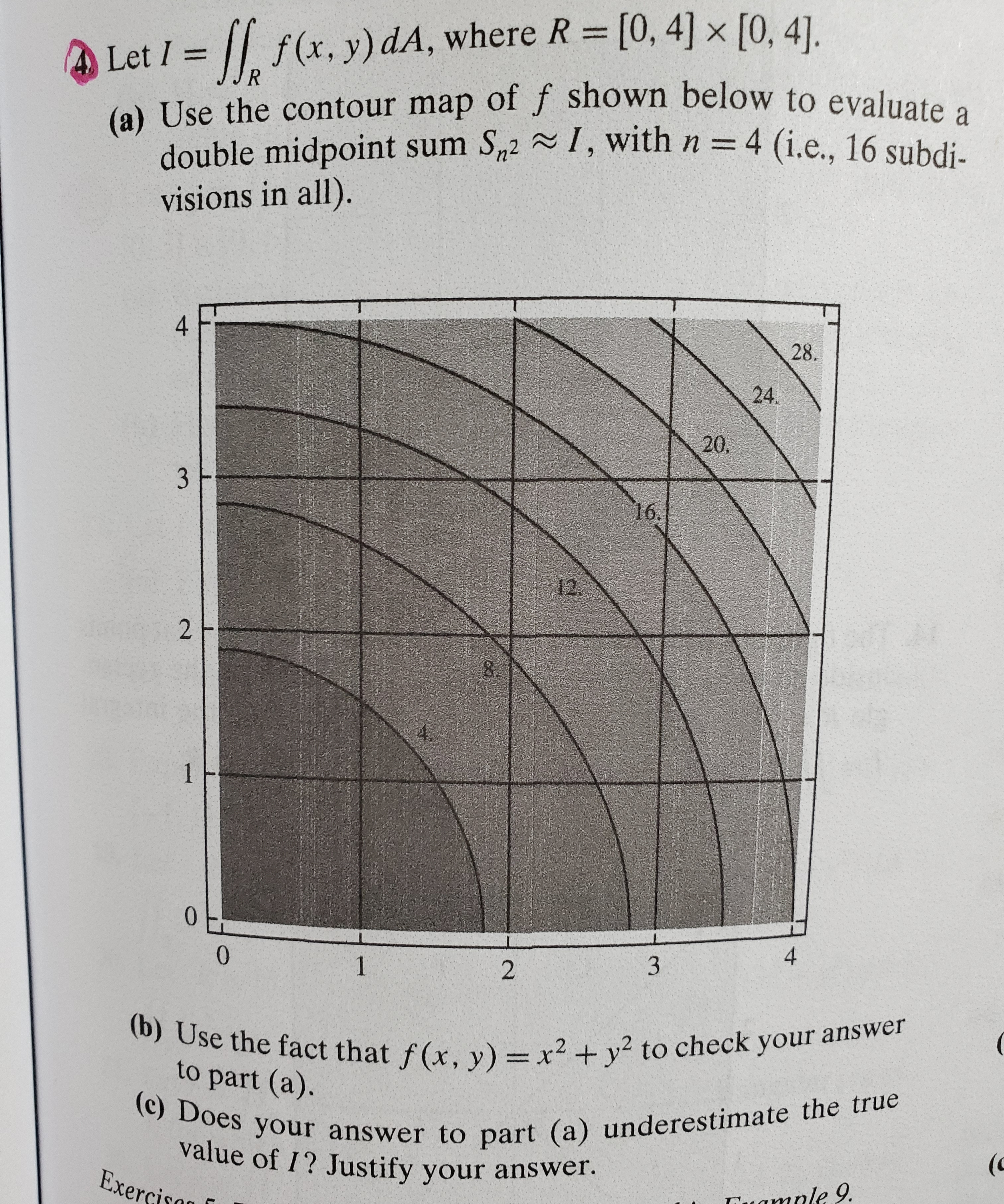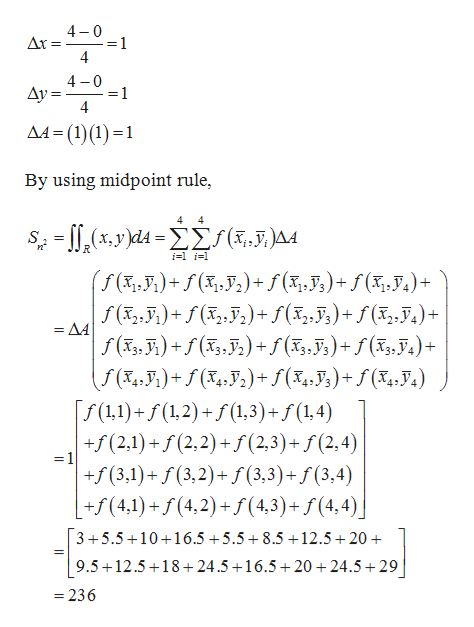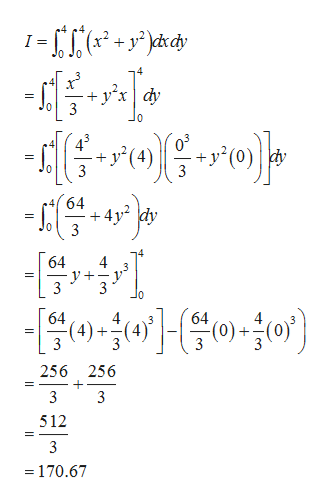# f(x, y) dA, where R [0, 4] x [0, 41].4 Let I=R(a) Use the contour map off shown below to evaluatedouble midpoint sum S2I, with n = 4 (i.e., 16 subdi.visions in all).428.24.20.316.1221004132(b) Use the fact that f(x, y) = x2 + y2 to check your answer(c) Does your answer to part (a) underestima te the trueto part (a).value of I? Justify your answer.Exercisan(camale 9.

Question
82 views

Question 4. Part a, b and c. Please!help_outlineImage Transcriptionclosef(x, y) dA, where R [0, 4] x [0, 41]. 4 Let I= R (a) Use the contour map off shown below to evaluate double midpoint sum S2I, with n = 4 (i.e., 16 subdi. visions in all). 4 28. 24. 20. 3 16. 12 2 1 0 0 4 1 3 2 (b) Use the fact that f(x, y) = x2 + y2 to check your answer (c) Does your answer to part (a) underestima te the true to part (a). value of I? Justify your answer. Exercisan (c amale 9. fullscreen
check_circle

Step 1

(a)

Given:

Step 2

Evaluate the function as follows.help_outlineImage Transcriptionclose4 0 Ar= =1 4 4 0 1 Ay= 4 AA (1)(11 By using midpoint rule, 4 4 -(,y)d4=S(,J.)]A4 x, ) i-1 i1 (f (%,,)+ f(x, J2)+ f (x,7,)+f(x,y,)+ f (X2, 7)+(,J)+f(3,,J,)+ f(,7.)+ 22. ΔΑ f (, )(,) +f (x,J,)+f(x,J,)+ f(X4, J) )+f(x, J;)+ f(x,,J.) f(11)+f(1,2)+f(1,3)+f(1,4) +f(2,1)+f(2,2)+f(2,3)+ f (2,4) 32 42. 42. =1 f (3,3)+ f (3,4) +f(3,1)(3,2)+ +f(4,1)+f (4,2)+f(4,3) + f (4,4)| 35.5 10 16.5+5.5+8.5+12.5 20 + = 9.5 12.5 18+24.5 16.5+ 20 24.5 29 236 fullscreen
Step 3

(b)

Evaluate the integra...help_outlineImage Transcriptionclose= + yxdy 3 43 + y(4) 3 64 4y dy 3 0 64 3 3 J0 64 4 4 (4) 3 (0) (4) (0): 256 256 3 3 512 3 = 170.67 fullscreen

### Want to see the full answer?

See Solution

#### Want to see this answer and more?

Solutions are written by subject experts who are available 24/7. Questions are typically answered within 1 hour.*

See Solution
*Response times may vary by subject and question.
Tagged in

### Other